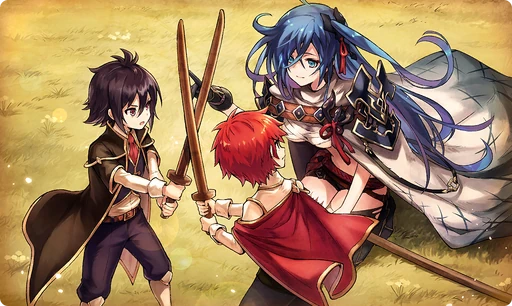# Future Sure to Come

Type
Equipment
Rank
5★
Max level
40
Enhancer cost
2000
Enhancer EXP
20000
Source
Unreleased
AlchemistCodeDBA distant dream, as I wandered the desert sands; I was looking upon a strange light with dubious eyes. I heard my own voice say, "Don't call me mistress. Not even teasingly, all right?" If the previous me knew of all I have avoided, she would doubtless have something to say about it. Yet the pain and the grief that come with holding a sword transform with the passage of time; that is the lesson I was taught by those two rays of light. That I was able to meet them is a small miracle that I still hold within my heart. (Setsuna's Thoughts)

## Stats

#### Simple stats

TypeLvl 1Lvl 30Lvl 40
HP+30+30+30

LevelHP
1+30
2+30
3+30
4+30
5+30
6+30
7+30
8+30
9+30
10+30
LevelHP
11+30
12+30
13+30
14+30
15+30
16+30
17+30
18+30
19+30
20+30
LevelHP
21+30
22+30
23+30
24+30
25+30
26+30
27+30
28+30
29+30
30+30
LevelHP
31+30
32+30
33+30
34+30
35+30
36+30
37+30
38+30
39+30
40+30

## Group skills

### Crowd Spirit

#### Simple stats

TypeLvl 1Lvl 30Lvl 40
PATK+10+10+10
PDEF+10+10+10
MATK+10+10+10
MDEF+10+10+10

#### Detailed stats

LevelPATKPDEFMATKMDEF
1+10+10+10+10
2+10+10+10+10
3+10+10+10+10
4+10+10+10+10
5+10+10+10+10
6+10+10+10+10
7+10+10+10+10
8+10+10+10+10
9+10+10+10+10
10+10+10+10+10
LevelPATKPDEFMATKMDEF
11+10+10+10+10
12+10+10+10+10
13+10+10+10+10
14+10+10+10+10
15+10+10+10+10
16+10+10+10+10
17+10+10+10+10
18+10+10+10+10
19+10+10+10+10
20+10+10+10+10
LevelPATKPDEFMATKMDEF
21+10+10+10+10
22+10+10+10+10
23+10+10+10+10
24+10+10+10+10
25+10+10+10+10
26+10+10+10+10
27+10+10+10+10
28+10+10+10+10
29+10+10+10+10
30+10+10+10+10
LevelPATKPDEFMATKMDEF
31+10+10+10+10
32+10+10+10+10
33+10+10+10+10
34+10+10+10+10
35+10+10+10+10
36+10+10+10+10
37+10+10+10+10
38+10+10+10+10
39+10+10+10+10
40+10+10+10+10